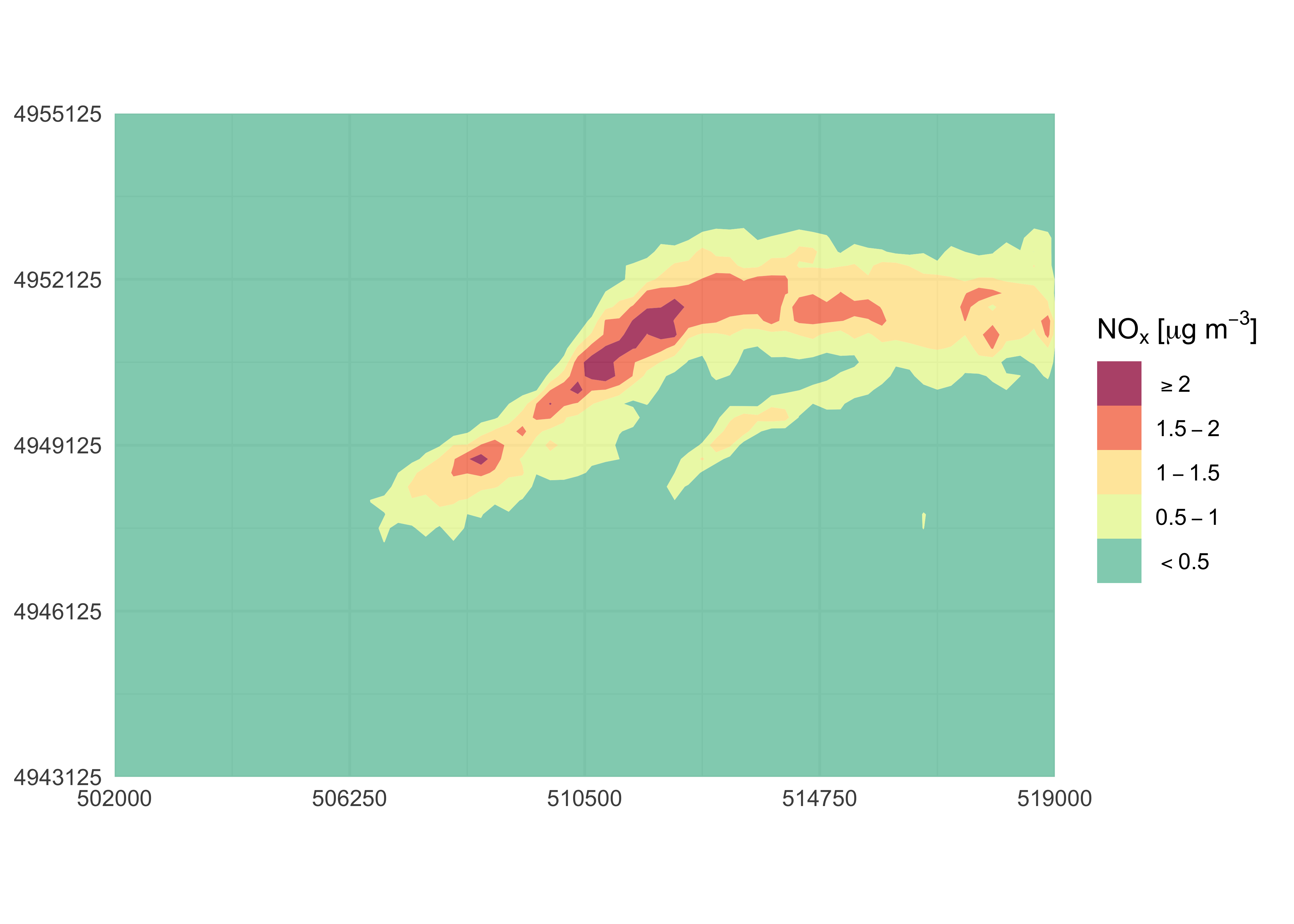# simulariatools

`simulariatools` is an open source collection of functions and tools useful to pre and post process data for air quality modelling and assessment. The package is developed and maintained by the people at Simularia and it is extensively used therein for daily jobs.

If you use this package in your work, please consider citing it. Refer to its Zenodo DOI to cite it.

## Installation

Installation of `simulariatools` from GitHub can be easily done using the `devtools` package:

``````    library("devtools")
install_github("Simularia/simulariatools")``````

The same commands can also be used to upgrade the package.

Note: in order to use `importADSOBIN()` to import ADSO/BIN data files, a working installation of Python 3 is required. For more information about R and Python interoperability, please refer to `reticulate` documentation.

## Brief examples

### Contour plot

Firt, import air quality data from NetCDF or ADSO/BIN files with the appropriate convenience function:

``````library(simulariatools)
mydata <- importRaster(file = "./test/conc_avg.nc",
k = 1000,
destaggering = TRUE,
variable = "nox",
verbose = TRUE)
#>
#> Raster statistics -----------------------------------------------
#>        X (min, max, dx)  :  496000.000   519250.000      250.000
#>        Y (min, max, dy)  : 4943000.000  4955250.000      250.000
#>      nox (min, max, mean):    0.00e+00     2.71e+00     1.52e-01
#> -----------------------------------------------------------------``````

A quick contour plot with default configuration can be easily obtained by running contourPlot2() without any argument:

``contourPlot2(mydata)``The plot is customisable by using contourPlot2() arguments and by piping ggplot2 instructions:

``````library(ggplot2)
contourPlot2(mydata,
domain = c(502000, 519000, 4943125, 4955125, 5, 5),
levels = c(-Inf, 0.5, 1, 1.5, 2, Inf),
legend = "NOx [ug/m3]") +
labs(x = NULL, y = NULL) +
theme_minimal()``````Use `ggsave()` to save the last plot to file:

``ggsave(filename = "~/path/to/myplot.png", width = 7, height = 6, dpi = 300)``

If a tile plot is required:

``````library(ggplot2)
contourPlot2(mydata,
tile = TRUE,
legend = "NOx [ug/m3]") +
labs(x = NULL, y = NULL) +
theme_minimal()``````## List of functions

Available functions are listed below:

• `contpourPlot2()`
• `downloadBasemap()`
• `importRaster()`.
• `importADSOBIN()`.
• `importSurferGrd()`.
• `plotAvgRad()`.
• `plotAvgTemp()`.
• `plotStabilityClass()`.
• `removeOutliers()`.
• `rollingMax()`.
• `stabilityClass()`.
• `vectorField()`.
• `contourPlot()` obsolete.
• `createBaseMap()` obsolete.

## Contact

Contact person:

``````Giuseppe Carlino
Simularia s.r.l.
g.carlino@simularia.it``````

## Contributors

Matteo Paolo Costa